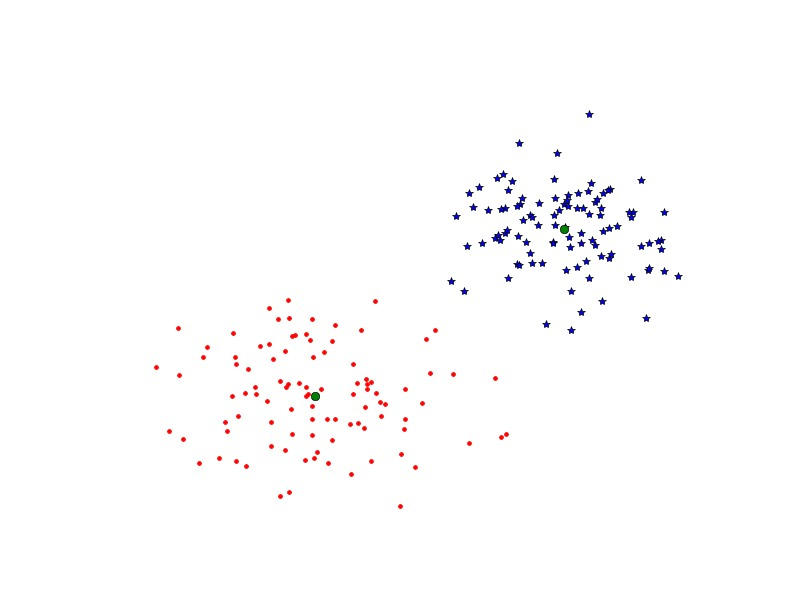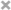K-means是聚类算法中最简单的一种聚类算法，它试着将输入数据划分成k簇，该算法最主要的缺点是需要事先选择聚类数目，并且如果选择不合理的话，聚类结果会很差。K-means以下面步骤迭代的提炼类中心：

• 初始化类中心，可以是随机的，也可以是估计的
• 将每个数据点分配给与它距离最近的类中心
• 对同属于一类的所有数据求平均，更新聚类中心

K-means试图最小化所有的within-class的方差。该算法是一种启发式提炼算法，在大多数情况下，它都很有效，但是并不能确保获得的解答是最好的。为了避免在初始化时选择不好而造成的影响，该算法通常会用不同的初始值运行几遍，然后选择方差最小的。虽然上面K-means的算法很容易实现，但由于有现成的实现vector quantization package,所以我们没必要再做自己去实现，直接使用上面的模块即可。

Example for numpy.vstack()：

>>> a = np.array([1, 2, 3])
>>> b = np.array([2, 3, 4])
>>> np.vstack((a,b)) 输出结果为：

array([[1, 2, 3], [2, 3, 4]])


>>> a = np.array([, , ])
>>> b = np.array([, , ])
>>> np.vstack((a,b)） 输出结果这里不给出了，具体可以再python shell上test。好了，现在我们了解了这个函数的作用，我们再来看scipy.cluster.vq()函数的作用，这里也直接给出实例，通过实例解释该函数的作用：


Example for scipy.cluster.vq():

>>> from numpy import array
>>> from scipy.cluster.vq import vq
>>> code_book = array([[1.,1.,1.],[2.,2.,2.]])
>>> features  = array([[  1.9,2.3,1.7],[  1.5,2.5,2.2],[  0.8,0.6,1.7]])
>>> vq(features,code_book)


(array([1, 1, 0]), array([ 0.43588989,  0.73484692,  0.83066239]))，


>>> from numpy import array
>>> from scipy.cluster.vq import vq
>>> code_book = array([[1.,1.,1.],[2.,2.,2.]])
>>> features  = array([[  1.9,2.3,1.7],[  1.5,2.5,2.2],[  0.8,0.6,1.7]])
>>> vq(features,code_book)
>>> from numpy import *
>>> dist = linalg.norm(code_book[1,:] - features[0,:])


"""
Function: Illustrate the k-means
Date: 2013-10-27
""""
from pylab import *
from scipy.cluster.vq import *
# 生成模拟数据
class1 = 1.5 * randn(100, 2)
class2 = randn(100,2) + array([5, 5])
features = vstack((class1,class2))
# K-Means聚类
centroids, variance = kmeans(features, 2)
code, distance = vq(features, centroids)
figure()
ndx = where(code==0)
plot(features[ndx,0], features[ndx,1],'*')
ndx = where(code==1)
plot(features[ndx, 0],features[ndx,1], 'r.')
plot(centroids[:, 0],centroids[:, 1], 'go')
axis('off')
show()